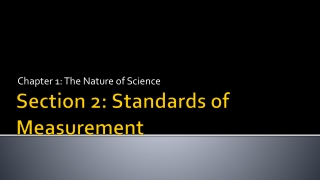Download PresentationSection 2: Standards of Measurement

# Section 2: Standards of Measurement

Télécharger la présentation## Section 2: Standards of Measurement

- - - - - - - - - - - - - - - - - - - - - - - - - - - E N D - - - - - - - - - - - - - - - - - - - - - - - - - - -
##### Presentation Transcript

1. Chapter 1: The Nature of Science Section 2: Standards of Measurement

2. Learning Goals • Name the prefixes used in SI and indicate what multiple of ten each one represents. • Identify SI units and symbols. • Convert related SI units.

3. Units and Standards • Standard: exact quantity that people agree to use for comparison.

4. Measurement Systems • Imperial system of measurement • Feet • Pounds • Gallons • Only 3 countries do not use the metric system of measurement: • U.S.A., Myanmar, and Liberia

5. Measurement Systems • International System of Units (SI): an improved version of the metric system. • Understood by scientists worldwide • Based on multiples of 10 and uses prefixes to indicate a specific multiple.

6. Measurement Systems • SI Base Units: • Length – meters (m) • Mass – grams (g) • Liquid volume – liters (L) • Time – seconds (s)

7. Measurement Systems • SI Prefixes: • Kilo (k) – x1000 • Centi (c) – x 0.01 or 1/100 • Milli (m) – x 0.001 or 1/1000 See the “Metric Toolbox” or Page 15 of your textbook for more

8. Measurement Systems • Converting between SI Units: • Use conversion factors: • ratios that are used to change one unit to another

9. Example • There are 1000 mL in 1 L. • How many mL are in 3 L?

10. Factor Label Method • Write what you know on the left • Write what you want on the right • Put a conversion factor in the middle • Cancel units on top and bottom • Multiply top and divide bottom

11. Example • There are 100 cm in 1 m. • How many cm are in 2.75 m?

12. Example • How long, in centimeters, is a 3,075 mm rope?

13. Let’s Practice!

14. Measuring Distance • Length is measured using a unit appropriate for the distance between two points.

15. Measuring Distance • The SI unit of length is the meter (m). mm cm

16. Measuring Volume • Volume: the amount of space an object occupies. • The base unit for liquid volume is liters (L) • Beakers, flasks, and graduated cylinders (mL)

17. Measuring Volume • Measuring solid volumes: • Formulas: (V = lxwxh) • Units in cubic centimeters (cm3) or cubic meters (m3) • Water displacement

18. Measuring Matter • Mass: a measurement of the quantity of matter in an object. • Base unit is grams (g) • Balances can be used to measure mass

19. Measuring Matter • Density: the mass per unit volume of a material. • How much mass an object has in a certain amount of space. • Density = mass/volume • Unit for density is grams per milliliter (g/mL)

20. Measuring Time and Temperature • SI unit of time = seconds (s) • Metric unit of temperature = degrees Celsius (°C) • SI unit of temperature = Kelvin (K)